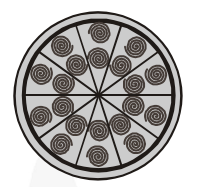Deepak Scored 45->99%ile with Bounce Back Crack Course. You can do it too!

# A brooch is made with silver wire in the form of a circle with diameter 35 mm.

Question:

A brooch is made with silver wire in the form of a circle with diameter 35 mm. The wire is also used in making 5 diameters which divide the circle into 10 equal sectors as shown in fig. Find:

(i) the total length of the silver wire required.

(ii) the area of each sector of the brooch.Solution:

Diameter of the circle = 35 mm

$\therefore \operatorname{Radius}(\mathrm{r})=\frac{\mathbf{3 5}}{\mathbf{2}} \mathrm{mm}$

(i) Circumference $=2 \pi \mathrm{r}$

$=2 \times \frac{22}{7} \times \frac{35}{2} \mathrm{~mm}=22 \times 5=110 \mathrm{~mm}$

Length of 1 piece of wire used to make diameter to divide the circle into

10 equal sectors = 35 mm

$\therefore \quad$ Length 5 pieces $=5 \times 35=175 \mathrm{~mm}$

$\therefore$ Total length of the silver wire

$=110+175 \mathrm{~mm}=285 \mathrm{~mm}$

(ii) Since the circle is divided into 10 equal sectors,

$\therefore$ Sector angle $\theta=\frac{\mathbf{3 6 0}^{\circ}}{\mathbf{1 0}}=\mathbf{3 6}^{\circ}$

$\Rightarrow$ Area of each sector

$=\frac{\theta}{360^{\circ}} \times \pi \mathrm{r}^{2}=\frac{36^{\circ}}{360^{\circ}} \times \frac{22}{7} \times \frac{35}{2} \times \frac{35}{2} \mathrm{~mm}^{2}$

$=\frac{\mathbf{1 1} \times \mathbf{3 5}}{\mathbf{4}} \mathrm{mm}^{2}=\frac{\mathbf{3 8 5}}{\mathbf{4}} \mathrm{mm}^{2}$